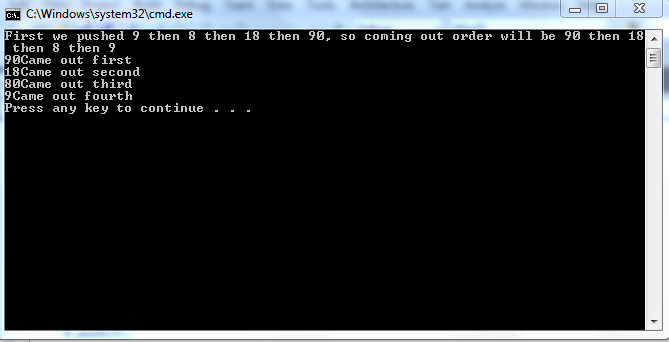# Stacks using c++ part2

In this tutorial, you will learn 1. When stacks should be implemented by arrays. 2. When stacks should be implemented by linked list. 3. Implementation of stacks using linked list. When should the stacks be implemented by linked list? In stacks, data is entered and removed from same end. So, there is no insertion and deletion from middle. As we learnt that while inserting data in the middle of an array, this creates a problem. Since in stacks, data is inserted and removed from one end only. So, if we know the number of elements that we have to insert in stack, we can use arrays. Using an array, we can save a lot of space because in case of arrays, you need an array,which takes a single memory space for each element, and a counter. While in case of linked list, you first have to make class of linked list consisting of nodes and each node occupies extra space of a pointer .Thus, by using an array, we can save space. If we don’t know the maximum amount of data we want in our stacks and we want to use arrays, we must build a very large array and this would again require a lot of space . Thus, while using array for implementation of stacks, maximum number of elements must be known. When should the stack be implemented by linked list ? Linked list can be used when the maximum number of elements we can have is unknown . Because linked list is a dynamic type of data structure so it can accommodate any amount of data if used properly. What is the implementation of stacks using linked list?
`class Stack{private:	linked_list l;public:	Stack(){}	void push(int value)	{		l.insert_beg(value);	}	bool Empty()	{		if(l. checkempty())			return 1;		return 0;	}	int get_top()	{		if(Empty())		{			cout<<"\n\tStack is Empty";			return 0;		}		cout<<"\nTop:\t"<<l.start()->info;		return l.start()->info; 	}	int pop()	{		if(Empty())		{			cout<<"\n\tStack is Empty";			return 0;		}		else 		{			int c=l.start()->info;			l.delete_beg();                     return c;		}	}};`
This is the implementation of stacks using linked list. Actually, first you have to make the linked list class discussed in last tutorials. Making a linked list class is necessary because you have to make an onject of data type linked list in the class of stacks. So, first we make an object of linked list in the class of stacks. The constructor is empty i.e. you don’t have to do any initialization when an object of stacks is made. ‘ The next function push and it is used for inserting data in the stack. We discussed it before that in case of stacks, data is inserted and removed from same end like dishes when we pile them up. So, we could have either used function to insert at start or the function to insert at end. But, we chose function to insert at start. This is because if you remember both insert at start and insert at end functions, the code for insertion at start was very precise and small. Hence, we can save some resources. Thus, insertion at start is widely used for linked list. Now, the next function is used to check whether, our stack is empty or not. In linked list we aso had such a function and we called it checkempty(). The checkempty() function simply checks whether the head of linked list is null or not. If, it is null then linked list is empty and if it is not NULL then the linked list is not empty. We simply call that function here. As data of stacks is being stored in linked list, if linked list is empty then automatically, stacks are empty. Now the next function is used to get the top value of stack. We don’t delete the data. We just print it and return it. This is quite useful when we need an idea of the thing on top. E.g. If, you were washing dishes, you want to know which dish is coming out before it actually does come out. The next function is pop function. It is used to return and delete the top element. Note: This conclude our stacks and in next tutorial, queues will be discussed. A code is also given in which there are small number of changes in class of linked list. SO do go through the C++ code. Output: